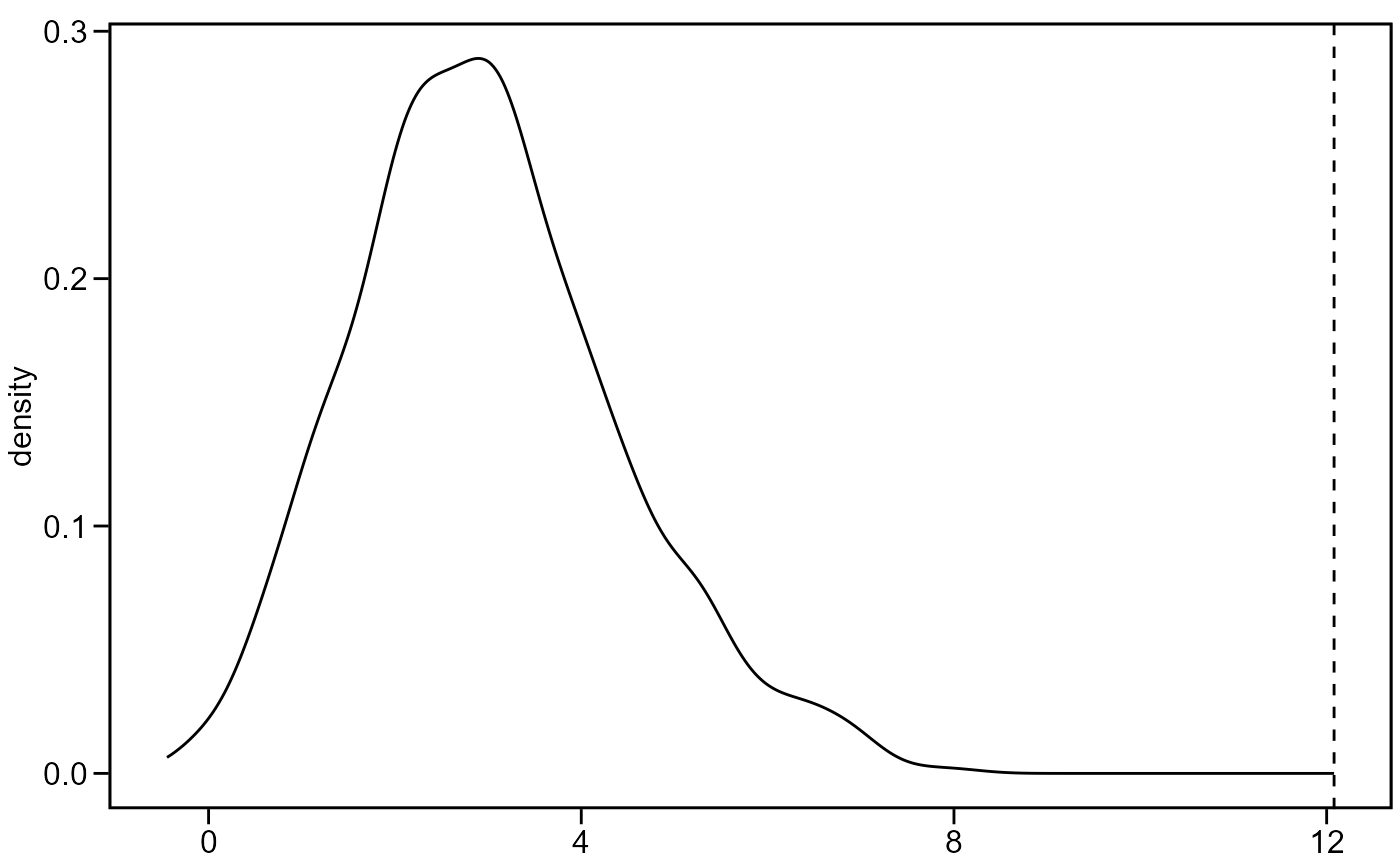Performs a Mantel test between two correlation/distance matrices. The function calculates the correlation between two matrices, the Z-score that is is the sum of the products of the corresponding elements of the matrices and a two-tailed p-value (null hypothesis: $r = 0$).

## Usage

mantel_test(mat1, mat2, nboot = 1000, plot = FALSE)

## Arguments

mat1, mat2

A correlation matrix or an object of class dist.

nboot

The number of permutations to be used. Defaults to 1000.

plot

if plot = TRUE, plots the density estimate of the permutation distribution along with the observed Z-score as a vertical line.

## Value

• mantel_r The correlation between the two matrices.

• z_score The Z-score.

• p-value The quantile of the observed Z-score. in the permutation distribution.

pairs_mantel()

## Author

Tiago Olivoto tiagoolivoto@gmail.com

## Examples

# \donttest{
library(metan)
# Test if the correlation of traits (data_ge2 dataset)
# changes between A1 and A2 levels of factor ENV
A1 <- corr_coef(data_ge2 %>% subset(ENV == "A1"))[["cor"]]
A2 <- corr_coef(data_ge2 %>% subset(ENV == "A2"))[["cor"]]
mantel_test(A1, A2, plot = TRUE)#> $mantel_r #>  0.7154291 #> #>$z_score
#>  12.07974
#>
#> \$p_value
#>  0.000999001
#>

# }